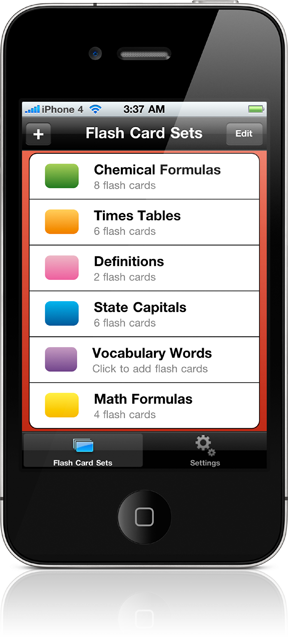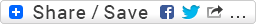Tuesday, March 26, 2019# Metric conversion calculator makes metric measurements easy

If you are travelling in a country that uses the metric systems, then you must face some of the difficulties while making some of the important and useful unit conversions, as it is impossible to remember all the units of conversions.

Thus, here is the easiest and the best way of converting the useful units without much calculation with the use of online metric conversions. This is very helpful as it is always available whenever you are in need. And you don’t even have to carry it with you, all you need is an internet connection and a computer or laptop or a smart phone.

Here are some of the most common and important type of conversions you usually need in your daily life:

Distance conversion

Distance conversion is very important at the moment when you are travelling in a country that uses the metric system. There are various units in a distance conversion, but the basic unit of measurement that can be compared with is the kilometre. A kilometreis roughly same as the 3/5 of mile; therefore one mile contains 1.6 kilometres. There is another unit for the measurement of distance that is the meter. A meter is known to be the little bit longer than a yard whereas a kilometre is equal to one thousand meters. Thus, the work of the online metric converter is to convert these units into the required one and tells you the correct answers instantly.

Volume conversion is also one of the important types of conversion that you must need while travelling to a country that uses the metric system. As the amount of fuel required to travel to a particular distance is usually measured in volumes. The basic metric unit for the volume is the litre. And all the country that uses the metric systems will provide you fuels in the litres only. A litre is calculated to be a bit more than a quart, and a gallon consists of 3.78 litres.

Weight conversions

Weight conversion is also very important type of conversion you might need when you are going to shopping of food or cooking as well as while using recipes that are written in metric units. The basic unit of the measurement of weight is the kilogram, where one kilogram is equal to 2.2 pounds. When you want to convert the unit to the smaller unit that is in grams, then you must remember that one kilogram is equal to one thousand grams or .022 pounds. This will help you providing the correct idea of amount of ingredients you want to purchase for your recipe.

So, as per discussed above the online metric conversions is very important for you in your day to day life as well as in many different and various fields of life. It helps you to get your answers of measurement conversions in seconds without performing any complex calculations just by using the online metric converters.